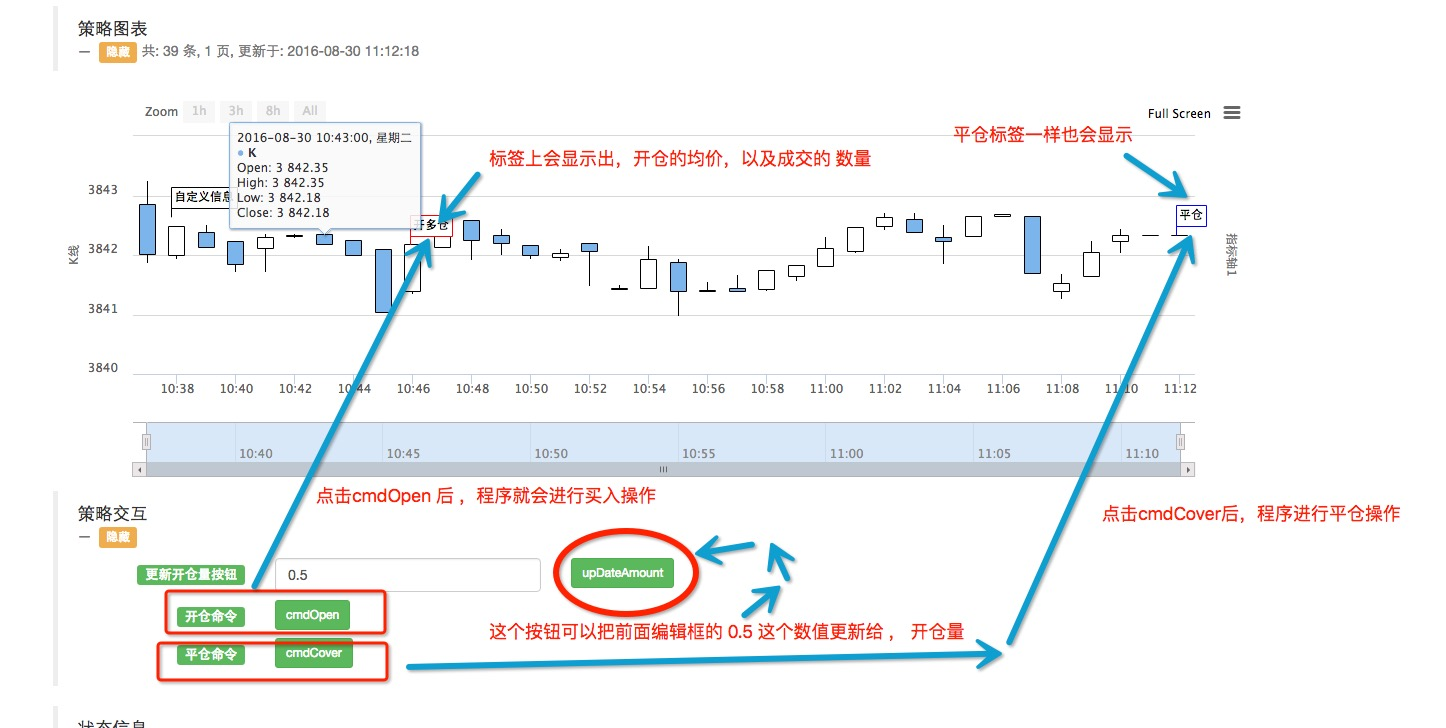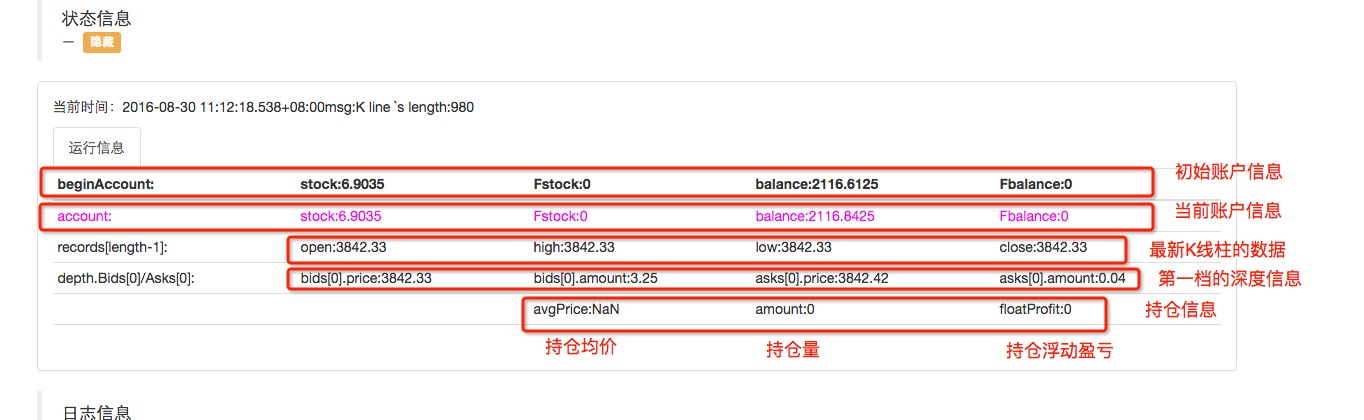# 简单的策略框架（可以自行修改扩展）

Author: 小小梦, Created: 2016-08-30 11:38:10, Updated: 2017-10-11 10:34:57

### 简单的策略框架（可以自行修改扩展）

• #### 说明:

• 1、 这个是个策略框架，没有触发开多仓、平仓的代码。 也就是说 策略开仓时机、平仓时机，开仓、平仓要满足的条件可以自行扩展。
• 2、 已具备收益模块，程序收益计算已经完成。 如有额外的需求，可以自行改动。
• 3、 该框架有界面交互功能，可以动态修改 开仓量（开仓币数）。可以手动下命令 开仓、平仓。如有需要额外的交互命令，可以阅读代码自行扩展。
• 4、 策略框架显示一个基本的K线图（使用了图表模板），策略框架的K线图上没有 指标线，如有需要，请自行扩展。（用法可以看图表模板）
• 5、 为了方便使用，策略框架使用了 数字货币交易类库 模板，如需自己写交易细节，可以在相应的位置修改。
• 6、 策略框架 运行时的数据显示在状态栏表格中，包括获取的行情数据、账户信息等，如需增加其他项目信息，可以自行扩展。• 策略框架源码：
``````//耐心看完会有收获的！
var Interval = 500;
var _long = 1;
var free = 0;
var state = free; //每次开仓   平仓  重置
var sellInfo = null; //每次开仓重置
var initAccount = null; //每次平仓重置
var beginAccount = null; //不重置
var Profit = 0; //已实现盈亏
var prefloatProfit = 0;//上次的浮动盈亏，滑动止盈 更新
var openBalance = 0;//开仓量
var isCover = false;

var tiaojian = 0; //这里可以设置  触发条件，比如 自定义的指标函数 发出开仓信号（比如返回了 数值  1 ）, 比如 0 时 是等待， 等于 2 时 是平仓
//也可能 触发了平仓条件
var Amount = 1;   //这里可以把 交易的量（币数）  写在程序里 自动控制（比如，根据盘口量）， 也可以设置在界面上做成界面上的参数，在程序运行的时候传进来。

var NowPositionInfo = {//持仓信息， 每次平仓更新
avgPrice: 0,
amount: 0 ,
floatProfit: 0
};

function openUpdate(){//开仓后的更新
state = _long;
sellInfo = null;
tiaojian = 0;//重置条件
}
function closeUpdate(){//平仓后的更新
state = free;
initAccount = _C(exchange.GetAccount);
NowPositionInfo.avgPrice = 0;
NowPositionInfo.amount = 0;
NowPositionInfo.floatProfit = 0;
isCover = true;
tiaojian = 0;//重置条件
}

function Calculate(nowAccount,nowDepth){//计算并更新收益 、 浮动收益 、计算 持仓均价 、持仓量
if(typeof(nowAccount) === 'undefined' ){
nowAccount = _C(exchange.GetAccount);
nowDepth = _C(exchange.GetDepth);
}
var diff_stocks = nowAccount.Stocks - initAccount.Stocks;//币之差
var diff_balance = nowAccount.Balance - initAccount.Balance;//钱之差
NowPositionInfo.avgPrice = Math.abs(diff_balance) / Math.abs(diff_stocks);
NowPositionInfo.amount = Math.abs(diff_stocks);
NowPositionInfo.floatProfit = diff_balance + diff_stocks * nowDepth.Bids.Price; //此次交易的浮动盈亏
Profit = (initAccount.Stocks - beginAccount.Stocks) * nowDepth.Bids.Price + (initAccount.Balance - beginAccount.Balance); //实现盈亏

//更新入界面
}

function get_Command(){//负责交互的函数，交互及时更新 相关数值 ，熟悉的用户可以自行扩展
var keyValue = 0;// 命令传来的参数 数值
var way = null; //路由
var cmd = GetCommand(); //获取  交互命令API
if (cmd) {
Log("按下了按钮：",cmd);//日志显示
arrStr = cmd.split(":"); // GetCommand 函数返回的 是一个字符串，这里我处理的麻烦了，因为想熟悉一下JSON ，所以先对字符串做出处理，把函数返回的字符串以 : 号分割成2个字符串。储存在字符串数组中。

if(arrStr.length === 2){//接受的不是 数值型的，是按钮型的。
jsonObjStr = '{' + '"' + arrStr + '"' + ':' + arrStr + '}'; // 把 字符串数组中的元素重新 拼接 ，拼接成 JSON 字符串  用于转换为JSON 对象。
jsonObj = JSON.parse(jsonObjStr); // 转换为JSON 对象

for(var key in jsonObj){ // 遍历对象中的  成员名
keyValue = jsonObj[key]; //取出成员名对应的 值 ， 就是交互按钮的值
}

if(arrStr == "upDateAmount"){// 此处为 数字型  。这里处理分为  按钮  和  数字型  。 详见 策略参数 设置界面 下的 交互设置
way = 1;
}
if(arrStr == "扩展1"){
way = 2;
}
if(arrStr == "扩展2"){
way = 3;
}
if(arrStr == "扩展3"){
way = 4;
}
}else if(arrStr.length === 1){// 此处为 按钮型
//路由
if(cmd == "cmdOpen"){
way = 0;
}
if(cmd == "cmdCover"){
way = 5;
}
}else{
throw "error:" + cmd + "--" + arrStr;
}
switch(way){ // 分支选择 操作
case 0://处理 发出开仓信号
tiaojian = 1;
break;
case 1://处理
Amount = keyValue;//把交互界面设置的 数值 传递给 Amount
Log("开仓量修改为：",Amount);//提示信息
break;
case 2://处理

break;
case 3://处理

break;
case 4://处理

break;
case 5://处理 发出平仓信号
tiaojian = 2;
break;
default: break;
}
}
}

function Loop(){//循环主体
//获取 行情、账户等信息
var account = _C(exchange.GetAccount);
var records = _C(exchange.GetRecords);
var depth = _C(exchange.GetDepth);
var len = records.length - 1;

//对获取的数据 容错处理
if(records.length < 10 ){//这里可以对 API 获取的数据 容错处理，这里举个例子 就是 获取的K线长度 必须大于10，小于10了  就返回 不做处理并在界面显示提示信息。
//输出到状态栏表格，显示K线长度不足
msg = "K线长度不足，获取中...";
return;
}
msg = "K line `s length:" + (len + 1);

//图表模板的使用---------------
\$.Draw(records);
//---------------------------

//第一次启动 处理的内容---------
if(isFirst === true){
\$.SignOP((new Date()).getTime(),null,null,3,"图表显示启动！");// 测试标记 自定义信息 到图表上
Log("程序启动！");
isFirst = false;
}
//--------------------------

//策略运行时状态栏表格上的显示数据 table.b1 就是往 b1 这个格子里 写入 "stock:" + account.Stocks + "#ff00ff"; 这些数据，可以对比截图，自己动手试试。
table.b1 = "stock:" + account.Stocks + "#ff00ff";
table.c1 = "Fstock:" + account.FrozenStocks + "#ff00ff";
table.d1 = "balance:" + account.Balance + "#ff00ff";
table.e1 = "Fbalance:" + account.FrozenBalance + "#ff00ff";
table.b2 = "open:" + records[len].Open;
table.c2 = "high:" + records[len].High;
table.d2 = "low:" + records[len].Low;
table.e2 = "close:" + records[len].Close;
table.b3 = "bids.price:" + depth.Bids.Price;
table.c3 = "bids.amount:" + depth.Bids.Amount;
table.c4 = "avgPrice:" + NowPositionInfo.avgPrice;
table.d4 = "amount:" + NowPositionInfo.amount;
table.e4 = "floatProfit:" + NowPositionInfo.floatProfit;
//-------------------------------------------------------------------------

//处理 策略交互
get_Command();//获取 并处理交互

//这里可以自定义  触发 操作的代码，比如 指标交叉了（当然这是你自定义的）， 就可以给 tiaojian 这个变量赋值 1， 即： tiaojian = 1; 这样下面满足条件就执行相应操作。

if(state === free && tiaojian === 1 ){//开仓条件，可以自行扩展，指标形态、差价、交易量 等等
//触发了上面的if括号内的条件，这个里面就是执行具体的开仓操作了，举个例子是用 数字货币交易类库 这个模板处理开仓。
return;
}
openUpdate();
}else if(state === _long && tiaojian === 2 ){
sellInfo = \$.Sell(NowPositionInfo.amount);
return;
}
\$.SignOP((new Date()).getTime(),sellInfo.price,sellInfo.amount,0);// 把操作标记 到图表上
closeUpdate();
}

//如果平仓了，更新收益-----------------------
Calculate();//计算收益，更新持仓状态
if(isCover === true){
LogProfit(Profit);
isCover = false;
}
//----------------------------------------

//更新图表---------------------------
\$.UpDateChart(records);
//---------------------------------
}

var table = null;
var msg = "";//显示在状态栏表格头部的 消息
var isFirst = true;

function main(){
//初始化
beginAccount = _C(exchange.GetAccount);//程序开始运行时的初始账户信息
initAccount = beginAccount;//每次开仓前的账户信息
table = \$.TableInit(5,6);
//初始化表格 5，代表 表格生成5列 分别是 a b c d e    ， 6代表表格生成 6行 分别是  0  1  2  3  4  5  6    。 这样最左上角的表格单元的坐标就是 a0 ,  table.a0 = 3; 此时就会显示到相应的表格

//给表格写上不许要变动的内容------------------
table.a1 = "account:" + "#ff00ff";
table.a2 = "records[length-1]:";
table.a0 = "beginAccount:";
table.b0 = "stock:" + beginAccount.Stocks;
table.c0 = "Fstock:" + beginAccount.FrozenStocks;
table.d0 = "balance:" + beginAccount.Balance;
table.e0 = "Fbalance:" + beginAccount.FrozenBalance;
//----------------------------------------------------

while(true){
Loop();//循环函数
\$.UpDateLogStatus(msg);//更新表格 数据
msg = "";
Sleep(Interval);
}
}
``````

More

Neo1898 loop和ontick有啥区别呢

kakababa2 梦总，给了启发！！超级棒！！

pengliheng 厉害了，我完全没看懂， 太长了， 先拿去用了，日后再爬坑

pengliheng ~_~一开始我觉得单纯的搬砖收益是不可计算的(动态的，会因为价格波动随时改变)，所以没去做这个搬砖收益的东西，后来发现基本上每个公开盘都有写纯搬砖收益。。。我就醉了。。。。。。。。。。。可能是我真的不会计算，我在想想